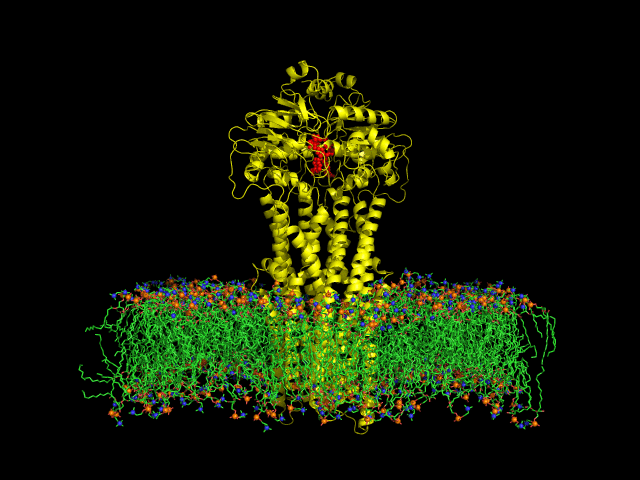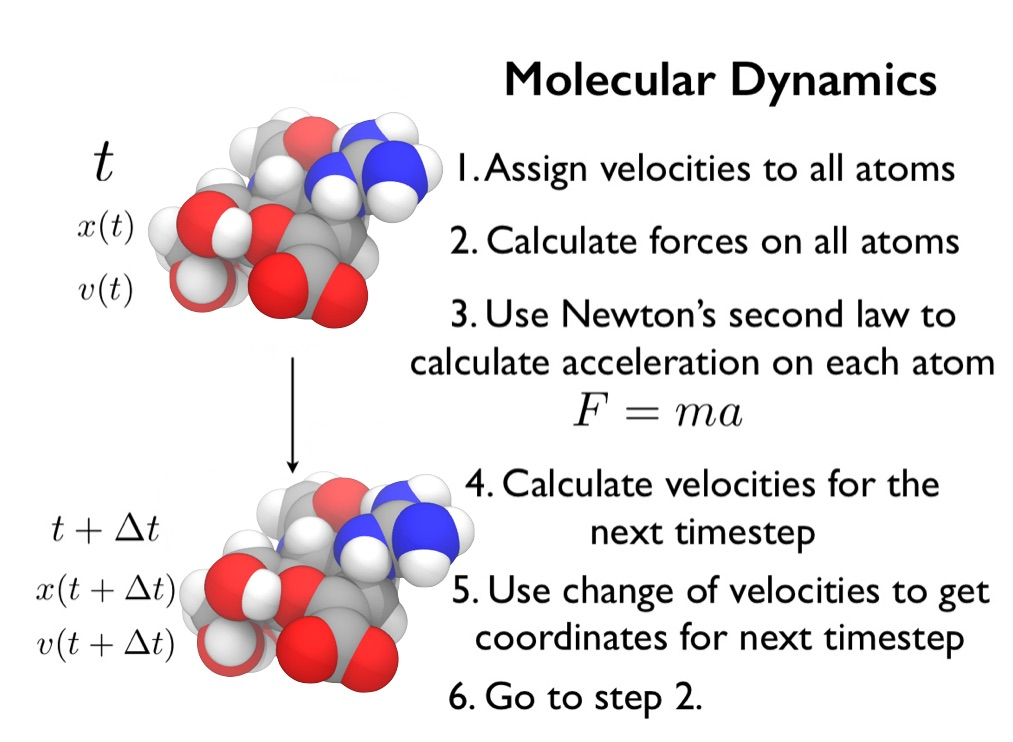## Molecular dynamics

cosmos 23rd January 2017 at 3:51pm
Biophysics Computational chemistry

Molecular dynamics (MD) simulations provide a computational microscope that lets you watch how a molecule moves over time.

MD works by calculating the forces that result from the interactions between atoms (e.g. electrostatic attraction and repulsion, interatomic van der Waals interactions, bonded interactions). These forces are calculated for every atom in each molecule in a molecular system, and used to work out how each atom would move over time. The result is a molecular dynamics trajectory, which can be used to produce a movie that approximates the motion of the complete biomolecular system.

Introduction to molecular dynamics simulation

http://www.chryswoods.com/dynamics/

VMD (molecular visualization)

http://www.nature.com/articles/srep03561

NAMD tutorialDIfferent levels of coarse-graining: from quantum to mesoscopic. These relate to length and time scales.

Thermostat, barostat.

## Force field.

Different water models.

Bond, angle, torsion, improper dihedral, van der Walls, electrostatic, ..

the particle mesh Ewald algorithm (PME). This is the clever algorithm that allows each periodic image to interact electrostatically with the infinite number of other periodic images. All simulations that use periodic boundary conditions should turn on PME.

### Simulating in thermodynamic ensembles

Note that the periodic boundary condition doesn't change the fact that a simulation where the (unit) volume is constant is a microcanonical ensemble. This is because the conditions required for the microcanonical ensemble are satisfied: the particle number, energy, and volume of the system are conserved (again, where the system is the unit volume, which we are simulating). If you think about it, reflecting boundary conditions and periodic b.c.s are not so different. The periodic one is just like the reflecting + teleportation to the opposite wall!

If we want to control the pressure, we need to use a barostat. Again, there are many different barostat algorithms available, with the choice of barostat normally coupled with the choice of thermostat. The best barostat to use for biomolecular simulations is namd is the “Langevin” barostat. This randomly changes the volume of the periodic box, and can be switched on by adding the following lines to ‘mdconfig’

Amber tools has many tools useful for MD simulations

A water-swap reaction coordinate for the calculation of absolute protein–ligand binding free energies

Steps

1. Minimisation. To remove any bad contacts between atoms that have been added (e.g. hydrogen atoms that have been added in a position that is too close to another atom of the protein), you first need to minimise the system. This involves calculating the force on all atoms, and then using this force to push the atoms towards stable, minimum energy configurations. The result will be a minimum energy conformation of the system.
2. Heating. The minimum energy conformation of the system is an approximation of the conformation that the molecules would adopt at near freezing conditions (near absolute zero kelvin). Molecular dynamics simulations of biomolecules are normally run at room temperature or body temperature. To prepare the system for simulation, you now have to slowly heat the molecules. This is to prevent the frozen atoms from exploding if you instantly ran the simulation at room (or body) temperature (imagine what happens if you throw an ice-cube into an extremely hot frying pan - I should add, you should not try this experiment at home as it can be dangerous).
3. Equilibration (NVT). The next step is to equilibrate the system using a short run of canonical (NVT) molecular dynamics. This will help the added water molecules find a stable (equilibrium) distribution around the protein and drug.
4. Equilibration (NPT). The final step is to equilibrate the system using a short run of isothermal-isobaric (NPT) molecular dynamics. This will adjust the box size, ensuring that the density of water in the periodic box is correct for the simulation temperature and pressure.
5. Production (NPT or NVT). The final step is the actual molecular dynamics simulation. This can be run either NPT or NVT depending on your choice (some people prefer NVT as it is quicker, and they argue that, once equilibrated, the volume will not change much). In our case, we will use NPT, and will combine steps 4 and 5 together.

http://www.chryswoods.com/dynamics/mutation/heating.html

Tutorial for using GROMACS for MD: link

## Free energy calculations

Meta-dynamics: bias the dynamics (using some descriptor coordinates), so that we explore the configuration space more. We then compute the free energy of the explored space by taking into account this bias.

These calculations can be used to guide Drug discovery